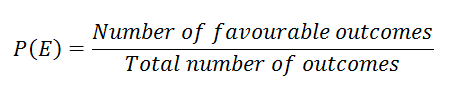# Chance And Probability

Suppose you go to watch a cricket match. When the match begins both the participating teams have an equal chance of winning. Slowly, when the match proceeds it becomes clearer which team will win the match. How do the people investing money in stock market decide to invest money in a particular stock? They take a risk-based on certain factors. Or the people who buy waiting tickets of train expecting it to get confirm they take a chance or risk.

## Chance and Probability of Events

There are many situations in real life where we have to take a chance or risk. Based on certain situations, the chance of occurrence of a certain event can be easily predicted. In simple words, the chance of occurrence of a particular event is what we study in probability.

Suppose you are playing a board game with your friends. On rolling a die, can you predict the result or can you get exactly the number of your choice? No, that is not possible. Such an experiment is known as a random experiment and the outcomes of this experiment are 1, 2, 3, 4, 5 and 6. Therefore experiments which do not have a fixed result are known as random experiments. The outcome of such experiments is uncertain and the result obtained after a random experiment has occurred is known as the outcome of that experiment. Each outcome of an experiment or a collection of outcomes make an event. If each outcome of an experiment has an equal chance of occurrence then these outcomes are equally likely. As in the example of rolling a die the chances of occurrence of numbers among 1 to 6 are equally likely.

## Probability Formula

The ratio of number of favorable outcomes to the number of total outcomes is defined as probability of occurrence of any event.Learn more about probability and statistics in an interesting way from here.